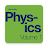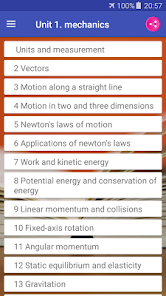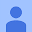# University Physics Volume 14.3
92 reviews
10K+EveryoneUniversity Physics is a three-volume collection that meets the scope and sequence requirements for two- and three-semester calculus-based physics courses. Volume 1 covers mechanics, sound, oscillations, and waves. Volume 2 covers thermodynamics, electricity and magnetism, and Volume 3 covers optics and modern physics. This textbook emphasizes connections between theory and application, making physics concepts interesting and accessible to students while maintaining the mathematical rigor inherent in the subject. Frequent, strong examples focus on how to approach a problem, how to work with the equations, and how to check and generalize the result.

* Complete Textbook by OpenStax
* Multiple Choices Questions (MCQ)
* Essay Questions Flash Cards
* Key-Terms Flash Cards

Unit 1. Mechanics
1. Units and Measurement

1.1. The Scope and Scale of Physics
1.2. Units and Standards
1.3. Unit Conversion
1.4. Dimensional Analysis
1.5. Estimates and Fermi Calculations
1.6. Significant Figures
1.7. Solving Problems in Physics
2. Vectors

2.1. Scalars and Vectors
2.2. Coordinate Systems and Components of a Vector
2.3. Algebra of Vectors
2.4. Products of Vectors
3. Motion Along a Straight Line

3.1. Position, Displacement, and Average Velocity
3.2. Instantaneous Velocity and Speed
3.3. Average and Instantaneous Acceleration
3.4. Motion with Constant Acceleration
3.5. Free Fall
3.6. Finding Velocity and Displacement from Acceleration
4. Motion in Two and Three Dimensions

4.1. Displacement and Velocity Vectors
4.2. Acceleration Vector
4.3. Projectile Motion
4.4. Uniform Circular Motion
4.5. Relative Motion in One and Two Dimensions
5. Newton's Laws of Motion

5.1. Forces
5.2. Newton's First Law
5.3. Newton's Second Law
5.4. Mass and Weight
5.5. Newton’s Third Law
5.6. Common Forces
5.7. Drawing Free-Body Diagrams
6. Applications of Newton's Laws

6.1. Solving Problems with Newton’s Laws
6.2. Friction
6.3. Centripetal Force
6.4. Drag Force and Terminal Speed
7. Work and Kinetic Energy

7.1. Work
7.2. Kinetic Energy
7.3. Work-Energy Theorem
7.4. Power
8. Potential Energy and Conservation of Energy

8.1. Potential Energy of a System
8.2. Conservative and Non-Conservative Forces
8.3. Conservation of Energy
8.4. Potential Energy Diagrams and Stability
8.5. Sources of Energy
9. Linear Momentum and Collisions

9.1. Linear Momentum
9.2. Impulse and Collisions
9.3. Conservation of Linear Momentum
9.4. Types of Collisions
9.5. Collisions in Multiple Dimensions
9.6. Center of Mass
9.7. Rocket Propulsion
10. Fixed-Axis Rotation

10.1. Rotational Variables
10.2. Rotation with Constant Angular Acceleration
10.3. Relating Angular and Translational Quantities
10.4. Moment of Inertia and Rotational Kinetic Energy
10.5. Calculating Moments of Inertia
10.6. Torque
10.7. Newton’s Second Law for Rotation
10.8. Work and Power for Rotational Motion
11. Angular Momentum

11.1. Rolling Motion
11.2. Angular Momentum
11.3. Conservation of Angular Momentum
11.4. Precession of a Gyroscope
12. Static Equilibrium and Elasticity

12.1. Conditions for Static Equilibrium
12.2. Examples of Static Equilibrium
12.3. Stress, Strain, and Elastic Modulus
12.4. Elasticity and Plasticity
13. Gravitation

13.1. Newton's Law of Universal Gravitation
13.2. Gravitation Near Earth's Surface
13.3. Gravitational Potential Energy and Total Energy
13.4. Satellite Orbits and Energy
13.5. Kepler's Laws of Planetary Motion
13.6. Tidal Forces
13.7. Einstein's Theory of Gravity
14. Fluid Mechanics

14.1. Fluids, Density, and Pressure
14.2. Measuring Pressure
14.3. Pascal's Principle and Hydraulics
14.4. Archimedes’ Principle and Buoyancy
14.5. Fluid Dynamics
14.6. Bernoulli’s Equation
14.7. Viscosity and Turbulence
Unit 2. Waves and Acoustics
15. Oscillations
16. Waves
17. Sound
Updated on
Mar 20, 2018

## Data safety

Safety starts with understanding how developers collect and share your data. Data privacy and security practices may vary based on your use, region, and age. The developer provided this information and may update it over time.No data shared with third partiesNo data collected
4.3
92 reviewsOctober 16, 2019
The app does not allow me to ask questions it keeps telling me technical error, can't connect, and I have downloaded the 3 app... Aside from these the app is very nice and educational
21 people found this review helpful
Did you find this helpful?